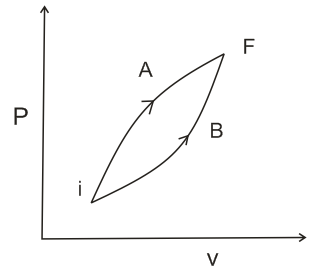# Following figure shows two processes A and B for a gas. If  and  are the amount of heat absorbed by the system in two cases, and  and  are changes in internal energies, respectively, then :Option 1)      Option 2) Option 3) Option 4)Internal energy is state function

So,

Now,

Now      (work is Area under the curve

So,

Option 1)

Option 2)

Option 3)

Option 4)

Exams
Articles
Questions